discuss high school / college related stuff here

Moderators: Kaitou, sxetnrdrmr, billc36, iDrum, Novak, PanasonicYouth

Page 2 of 2 [ 32 posts ]
Message
Author
noname Offline
ROAF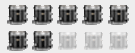Posts: 1852
Joined: Tue May 06, 2008 4:14 pm

Posted: Sun Nov 28, 2010 8:33 pm

tommyservo7 wrote:
noname wrote: 2x+2z=2
5x+3y=4
3y-4z=4
The textbook says the solution should be (-4,8,5). How do you get this answer? I know it's something really simple, but I can't remember how to do it.
I tried. Looks like it requires a matrix.

EDIT: This nifty little thing works excellently. It will teach you how to do it.
Thanks for the help. If this is the way to go, thank you, but I don't this is how it was taught in class. If anyone else has an alternative method, please let me know.

AWA Offline
MAN Chops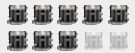Posts: 3939
Joined: Sun Jan 04, 2009 1:35 pm
Location: West Deptford, New Jersey, The United States of America, Earth, The Solar System, The Milky Way

Posted: Sun Nov 28, 2010 8:51 pm

noname wrote: 2x+2z=2
5x+3y=4
3y-4z=4
The textbook says the solution should be (-4,8,5). How do you get this answer? I know it's something really simple, but I can't remember how to do it.
2x+2z=2
5x+3y=4
3y-4z=4
It's a system of equations.

The first equation (solved for z):
z=1-x

Substitute this statement into the third equation:
3y-4(1-x)=4
3y-4+4x=4
3y+4x=8

Now you have a simple system of two variables. Solve with the second equation (equation addition and subtraction):
3y+4x=8
3y+5x=4
-x=4
x=-4

Now substitute this x-value into the first equation:

2(-4)+2z=2
-8+2z=2
2z=10
z=5

You may choose to use either the x-value or the z-value to solve for y. Let us use the z-value for variety:
3y-4(5)=4
3y-20=4
3y=24
y=8

Putting our three results into an ordered triple:
(x,y,z)
(-4,8,5)

2007 - 2010: West Deptford High School (07-08 FE, 09-10 quads)
2013: Spirit of Atlanta (Marimba)
2014: Bluecoats (Vibraphone)

-Glenn Dawson

"Pedicabo ego vos et irrumabo."

noname Offline
ROAFPosts: 1852
Joined: Tue May 06, 2008 4:14 pm

Posted: Sun Nov 28, 2010 9:06 pm

AWA wrote:
noname wrote: 2x+2z=2
5x+3y=4
3y-4z=4
The textbook says the solution should be (-4,8,5). How do you get this answer? I know it's something really simple, but I can't remember how to do it.
2x+2z=2
5x+3y=4
3y-4z=4
It's a system of equations.

The first equation (solved for z):
z=1-x

Substitute this statement into the third equation:
3y-4(1-x)=4
3y-4+4x=4
3y+4x=8

Now you have a simple system of two variables. Solve with the second equation (equation addition and subtraction):
3y+4x=8
3y+5x=4
-x=4
x=-4

Now substitute this x-value into the first equation:

2(-4)+2z=2
-8+2z=2
2z=10
z=5

You may choose to use either the x-value or the z-value to solve for y. Let us use the z-value for variety:
3y-4(5)=4
3y-20=4
3y=24
y=8

Putting our three results into an ordered triple:
(x,y,z)
(-4,8,5)

That's more like what we learned in class. Thanks to both of you for your help.

waffley Offline
chops master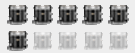Posts: 1266
Joined: Tue May 11, 2010 2:59 am

Posted: Fri Feb 04, 2011 3:57 am

My homework will be due before anyone sees this, but here's a couple questions.

This one's multiple choice:
The average velocity of an object for a given motion points to the right. When is it possible for the instantaneous velocity of the object to point to the left? (Select all that apply.)
-at the beginning of the motion
-at some point in the middle of the motion
-at the end of the motion

I just 100% don't get this question and so I got it wrong.

Also this:

You drive in a straight line at 18.0 m/s for 12.0 miles, then at 30.0 m/s for another 12.0 miles.
(a) How does your average speed compare with 24.0 m/s?
speedav < 24.0 m/s
speedav > 24.0 m/s
speedav = 24.0 m/s
Explain.

I originally was like "oh I'm traveling different speeds over the same distance so I can just add those and take the average of that" which ended up being wrong. Later on in the question you calculate the average velocity and I realized that since you are traveling the same distance with different speeds, the time in which you travel those distances is different so you can't really take a shortcut there and you have to actually calculate out the distance traveled over time. Right? Does that make sense?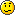DCI open summer diddle band '11 '12
DCI world summer diddle band '13
WGI world winter band '12 '14
HS teachin' band '12-'15

ottomagne Offline
MAN ChopsPosts: 3114
Joined: Mon Sep 14, 2009 1:24 am
Location: Houston, TX

Posted: Fri Feb 04, 2011 11:30 am

waffley wrote:My homework will be due before anyone sees this, but here's a couple questions.

This one's multiple choice:
The average velocity of an object for a given motion points to the right. When is it possible for the instantaneous velocity of the object to point to the left? (Select all that apply.)
-at the beginning of the motion
-at some point in the middle of the motion
-at the end of the motion

I just 100% don't get this question and so I got it wrong.

Also this:

You drive in a straight line at 18.0 m/s for 12.0 miles, then at 30.0 m/s for another 12.0 miles.
(a) How does your average speed compare with 24.0 m/s?
speedav < 24.0 m/s
speedav > 24.0 m/s
speedav = 24.0 m/s
Explain.

I originally was like "oh I'm traveling different speeds over the same distance so I can just add those and take the average of that" which ended up being wrong. Later on in the question you calculate the average velocity and I realized that since you are traveling the same distance with different speeds, the time in which you travel those distances is different so you can't really take a shortcut there and you have to actually calculate out the distance traveled over time. Right? Does that make sense?I didn't quite understand the first one, but I do get the second one.

18 m/s = 12/t1, 12/18 s = t1
30 m/s = 12/t2, 12/30 s = t2

Average velocity = dd/dt, or 24/t1+t2

Because t1+t2 > 1 (12/18 is 2/3, 12/30 > 10/30 = 1/3), we know that the average velocity that you traveled will be < 24 m/s.Drum bandin' since twenty o' seven.

'10 '13
'11'13

waffley Offline
chops masterPosts: 1266
Joined: Tue May 11, 2010 2:59 am

Posted: Sun Mar 20, 2011 10:18 pm

Posting this in here because everyone should know about this awesome guy who should probably be a tenured professor at some awesome university.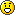DCI open summer diddle band '11 '12
DCI world summer diddle band '13
WGI world winter band '12 '14
HS teachin' band '12-'15

petrolika Offline
ramming notes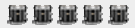Posts: 412
Joined: Sun Sep 25, 2011 5:35 am
Location: The Land of Oz.

Posted: Fri Sep 30, 2011 10:44 pm

Anyone here know anything about Agriculture, and knows how to do DSE (Dry Sheep Equivalent)??
Les femmes françaises ne sont pas poilu, ils ont juste beaucoup d'ombre autour de leurs aisselles, qui donne aux gens la perception de la pilosité français.

Page 2 of 2 [ 32 posts ]#### Who is online

Users browsing this forum: No registered users and 1 guest

 [phpBB Debug] PHP Warning: in file [ROOT]/vendor/twig/twig/lib/Twig/Extension/Core.php on line 1266: count(): Parameter must be an array or an object that implements Countable Jump to You cannot post new topics in this forumYou cannot reply to topics in this forumYou cannot edit your posts in this forumYou cannot delete your posts in this forumYou cannot post attachments in this forum